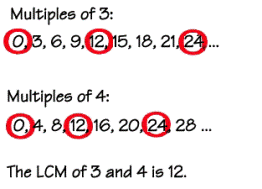# Least common multiple

The least common multiple of two numbers is the smallest positive integer that is the multiple of both numbers in question. It is also called the lowest common multiple or the smallest common multiple.
Example: It is necessary to find the least common multiple of numbers 6 and 8. The multiples of the number 6 are 6, 12, 18, 24, 30, 36, 42, 48…, while the multiples of the number 8 are 8, 16, 24, 32, 40, 48, 56… The common multiples of the two numbers are 24 and 48. The lesser of the two is 24 and therefore 24 is the least common multiple of the numbers 6 and 8. There is also a method to calculate the LCM using prime numbers, similar to the method used to find the greatest common factor, but it is less effective for finding the LCM. The usefulness of the LCM is the most apparent while adding, subtracting or comparing fractions. Finding the LCM of the denominators you are currently calculating with (also called the lowest common denominator) enables you to express the fractions in question as a fraction of the LCM. In other words, all of the fractions can be expressed as fractions of the same denominator, which is the lowest common denominator of all the denominators.The least common multiple can also be calculated for rational numbers while using the same principle.
Example: We need to calculate the least common multiple of the rational numbers 2/5 and 4/7. The multiples of the number 2/5 are 4/5, 6/5, 8/5, 10/5, 12/5, 14/5, 16/5, 18/5, 20/5… and the multiples of the number 4/7 are 4/7, 8/7, 12/7, 16/7, 20/7, 24/7, 28/7…, their least common multiple is 4 (20/5=28/7=4).
Knowledge of the least common multiple of two or more numbers is very useful while adding, subtracting or comparing fractions that have different denominators.

## Least common multiple exams for teachers

 Exam Name File Size Downloads Upload date Integers Least common multiple of integers – very easy 551.6 kB 5060 September 3, 2019 Least common multiple of integers – easy 558 kB 4898 September 3, 2019 Least common multiple of integers – medium 548.9 kB 5132 September 3, 2019 Least common multiple of integers – hard 550.4 kB 3389 September 3, 2019 Least common multiple of integers – very hard 553.8 kB 3084 September 3, 2019 Monomials Least common multiple of monomials – very easy 573.9 kB 2897 September 3, 2019 Least common multiple of monomials – easy 582.5 kB 2504 September 3, 2019 Least common multiple of monomials – medium 574.5 kB 2969 September 3, 2019 Least common multiple of monomials – hard 552.9 kB 2546 September 3, 2019 Least common multiple of monomials – very hard 550.5 kB 2736 September 3, 2019

## Least common multiple worksheets for students

 Worksheet Name File Size Downloads Upload date Least common multiple of integers 154.6 kB 4313 September 3, 2019 Least common multiple of monomials 277 kB 3668 September 3, 2019